Tuesday, April 16, 2013

Binary Search in Recursion : JAVA OOP

Write Java Source Code: Binary Search in Recursion

Sample Output 1:
Enter the size of the array: 5
Enter 5 numbers: 2 1 3 5 4
The sorted numbers are:
1 2 3 4 5
Enter the number you want to search: 3
The search number is on the index 2
Source Code:

//Java Class
public class binarySearch //Example of Java Class
{
//biSearch is an example of a method or a function
public int binSearch(int[] arr, int fIndex, int lIndex,int search)
{
int middle = (fIndex + (lIndex - fIndex) / 2);
if(fIndex<lIndex ){
if (search == arr[middle]){
return middle;
}
else if(search < arr[middle]){
if(search == arr)
return 0;
return binSearch(arr, fIndex, middle, search);
}
else if(search > arr[middle]){
if(search == arr[middle+1])
return middle + 1;
return binSearch(arr, middle+1, lIndex, search);
}
}
return -1;
}
//this is also a class method
public void sort(int[] arr)
{
for(int i=0; i<arr.length; i++)
{
for(int j=i+1; j<arr.length; j++ )
{
if(arr[i] > arr[j])
{
int temp = arr[j];
arr[j]=arr[i];
arr[i]= temp;
}
}
}
for(int i=0; i<arr.length; i++)
{
System.out.print(arr[i] + " ");
}
}
}
//main class
import java.util.Scanner;
public class Main {
public static void main(String[] args)
{
Scanner input = new Scanner(System.in);
System.out.print("Enter the size of the array: ");
int n = input.nextInt();
int[] x = new int[n];
System.out.print("Enter "+ n +" numbers: ");
int middle;
for(int i=0; i<n; i++)
{
x[i] = input.nextInt();
}
binarySearch access = new binarySearch(); //this is how to instantiate an object to access a class
System.out.println("The sorted numbers are: ");
access.sort(x);//this is how to access a method
System.out.println();

System.out.print("Enter the number you want to search: ");
int value = input.nextInt();
System.out.print("The search number is on the index ");
System.out.print(access.binSearch(x, 0, x.length-1, value)); //how to access a class
}
}

1.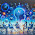Mohammad Ashraful Hasan Sobuj
My Batch- 46th
My Department :CSE
My ID 201420656

public class binarySearch
{
public int binSearch(int[] a, int fIndex, int lIndex,int search);
int m = (fIndex + (lIndex - fIndex) / 2);
if(fIndex a[m]);
{
if(search == a[m+1]);
return m + 1;
return binSearch(a, m+1, lIndex, search);
}
}
return -1;
}
public void sort(int[] a);
{
for(int i=0; i a[j]);
int temp = a[j];
a[j]=a[i];
a[i]= temp;
}
}
}
for(int i=0; i<a.length; i++);
{
System.out.print(a[i] + " ");
}
}
}
import java.util.Scanner;
public class Main
{
public static void main(String[] args);
{
Scanner input = new Scanner(System.in);
System.out.print("Enter the size of the array: ");
int n = input.nextInt();
int[] x = new int[n];
System.out.print("Enter "+ n +" numbers: ");
int m;
for(int i=0; i<n; i++);
{
x[i] = input.nextInt();
}
binarySearch access = new binarySearch();
System.out.println("The sorted numbers are: ");
access.sort(x);
System.out.println();
System.out.print("Enter the number you want to search: ");
int value = input.nextInt();
System.out.print("The search number is on the index ");
System.out.print(access.binSearch(x, 0, x.length-1, value));
}
}

2.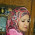sultana razia khan
My Batch- 46th
My Department :CSE
My ID 201420715

public class binarySearch
{
public int binSearch(int[] a, int fIndex, int lIndex,int search);
int m = (fIndex + (lIndex - fIndex) / 2);
if(fIndex a[m]);
{
if(search == a[m+1]);
return m + 1;
return binSearch(a, m+1, lIndex, search);
}
}
return -1;
}
public void sort(int[] a);
{
for(int i=0; i a[j]);
int temp = a[j];
a[j]=a[i];
a[i]= temp;
}
}
}
for(int i=0; i<a.length; i++);
{
System.out.print(a[i] + " ");
}
}
}
import java.util.Scanner;
public class Main
{
public static void main(String[] args);
{
Scanner input = new Scanner(System.in);
System.out.print("Enter the size of the array: ");
int n = input.nextInt();
int[] x = new int[n];
System.out.print("Enter "+ n +" numbers: ");
int m;
for(int i=0; i<n; i++);
{
x[i] = input.nextInt();
}
binarySearch access = new binarySearch();
System.out.println("The sorted numbers are: ");
access.sort(x);
System.out.println();
System.out.print("Enter the number you want to search: ");
int value = input.nextInt();
System.out.print("The search number is on the index ");
System.out.print(access.binSearch(x, 0, x.length-1, value));
}
}

3.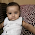This comment has been removed by the author.

4.Sharmin Akter
My Batch- 46th
My Department :CSE
My ID 201420769

public class binarySearch
{
public int binSearch(int[] a, int fIndex, int lIndex,int search);
int m = (fIndex + (lIndex - fIndex) / 2);
if(fIndex a[m]);
{
if(search == a[m+1]);
return m + 1;
return binSearch(a, m+1, lIndex, search);
}
}
return -1;
}
public void sort(int[] a);
{
for(int i=0; i a[j]);
int temp = a[j];
a[j]=a[i];
a[i]= temp;
}
}
}
for(int i=0; i<a.length; i++);
{
System.out.print(a[i] + " ");
}
}
}
import java.util.Scanner;
public class Main
{
public static void main(String[] args);
{
Scanner input = new Scanner(System.in);
System.out.print("Enter the size of the array: ");
int n = input.nextInt();
int[] x = new int[n];
System.out.print("Enter "+ n +" numbers: ");
int m;
for(int i=0; i<n; i++);
{
x[i] = input.nextInt();
}
binarySearch access = new binarySearch();
System.out.println("The sorted numbers are: ");
access.sort(x);
System.out.println();
System.out.print("Enter the number you want to search: ");
int value = input.nextInt();
System.out.print("The search number is on the index ");
System.out.print(access.binSearch(x, 0, x.length-1, value));
}
}

5.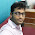Name : jalis Mahmud
ID : 201420762
Batch : 46th

public class binarySearch
{
public int binSearch(int[] a, int fIndex, int lIndex,int search);
int x = (fIndex + (lIndex - fIndex) / 3);
if(fIndex a[x]);
{
if(search == a[x+2]);
return x + 2;
return binSearch(a, x+2, lIndex, search);
}
}
return -2;
}
public void sort(int[] a);
{
for(int i=0; i a[j]);
int temp = a[j];
a[j]=a[i];
a[i]= temp;
}
}
}
for(int i=0; i<a.length; i++);
{
System.out.print(a[i] + " ");
}
}
}
import java.util.Scanner;
public class Main
{
public static void main(String[] args);
{
Scanner input = new Scanner(System.in);
System.out.print("Enter the size of the array: ");
int n = input.nextInt();
int[] x = new int[n];
System.out.print("Enter "+ n +" numbers: ");
int m;
for(int i=0; i<n; i++);
{
x[i] = input.nextInt();
}
binarySearch access = new binarySearch();
System.out.println("The sorted numbers are: ");
access.sort(x);
System.out.println();
System.out.print("Enter the number you want to search: ");
int value = input.nextInt();
System.out.print("The search number is on the index ");
System.out.print(access.binSearch(x, 0, x.length-1, value));
}
}

6.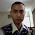ID-201420406
Batch-46th

public class binarySearch
{
public int binSearch(int[] a, int fIndex, int lIndex,int search);
int m = (fIndex + (lIndex - fIndex) / 2);
if(fIndex a[m]);
{
if(search == a[m+1]);
return m + 1;
return binSearch(a, m+1, lIndex, search);
}
}
return -1;
}
public void sort(int[] a);
{
for(int i=0; i a[j]);
int temp = a[j];
a[j]=a[i];
a[i]= temp;
}
}
}
for(int i=0; i<a.length; i++);
{
System.out.print(a[i] + " ");
}
}
}
import java.util.Scanner;
public class Main
{
public static void main(String[] args);
{
Scanner input = new Scanner(System.in);
System.out.print("Enter the size of the array: ");
int n = input.nextInt();
int[] x = new int[n];
System.out.print("Enter "+ n +" numbers: ");
int m;
for(int i=0; i<n; i++);
{
x[i] = input.nextInt();
}
binarySearch access = new binarySearch();
System.out.println("The sorted numbers are: ");
access.sort(x);
System.out.println();
System.out.print("Enter the number you want to search: ");
int value = input.nextInt();
System.out.print("The search number is on the index ");
System.out.print(access.binSearch(x, 0, x.length-1, value));
}
}

7.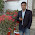import java.util.Scanner;

public class binarySearch
public int binSearch(int[] arr, int fIndex, int lIndex,int search)
{

int middle = (fIndex + (lIndex - fIndex) / 2);

if(fIndex arr[middle]){
if(search == arr[middle+1])
return middle + 1;
return binSearch(arr, middle+1, lIndex, search);
}

}
return -1;
}

public void sort(int[] arr)
{
for(int i=0; i arr[j])
{
int temp = arr[j];
arr[j]=arr[i];
arr[i]= temp;
}
}
}

for(int i=0; i<arr.length; i++)
{
System.out.print(arr[i] + " ");
}
}
}

import java.util.Scanner;

public class Main {

public static void main(String[] args)
{
Scanner input = new Scanner(System.in);

System.out.print("Enter the size of the array: ");
int n = input.nextInt();
int[] x = new int[n];

System.out.print("Enter "+ n +" numbers: ");
int middle;
for(int i=0; i<n; i++)
{
x[i] = input.nextInt();
}

binarySearch access = new binarySearch();
System.out.println("The sorted numbers are: ");
access.sort(x);
System.out.println();

System.out.print("Enter the number you want to search: ");
int value = input.nextInt();

System.out.print("The search number is on the index ");
System.out.print(access.binSearch(x, 0, x.length-1, value));
}
}

8.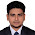ID-201420763
Batch-46th

public class binarySearch
{
public int binSearch(int[] a, int fIndex, int lIndex,int search);
int m = (fIndex + (lIndex - fIndex) / 2);
if(fIndex a[m]);
{
if(search == a[m+1]);
return m + 1;
return binSearch(a, m+1, lIndex, search);
}
}
return -1;
}
public void sort(int[] a);
{
for(int i=0; i a[j]);
int temp = a[j];
a[j]=a[i];
a[i]= temp;
}
}
}
for(int i=0; i<a.length; i++);
{
System.out.print(a[i] + " ");
}
}
}
import java.util.Scanner;
public class Main
{
public static void main(String[] args);
{
Scanner input = new Scanner(System.in);
System.out.print("Enter the size of the array: ");
int n = input.nextInt();
int[] x = new int[n];
System.out.print("Enter "+ n +" numbers: ");
int m;
for(int i=0; i<n; i++);
{
x[i] = input.nextInt();
}
binarySearch access = new binarySearch();
System.out.println("The sorted numbers are: ");
access.sort(x);
System.out.println();
System.out.print("Enter the number you want to search: ");
int value = input.nextInt();
System.out.print("The search number is on the index ");
System.out.print(access.binSearch(x, 0, x.length-1, value));
}
}

9.Once a person has decided which website or websites to work with, he or she will need to determine how much money should be invested in binary options.my company

10.11.12.Overall, the Ultimate Profit Solution is an amazing manual forex trading system. There are many videos on the Ultimate Profit Solution website where you can see it in action and get a feel for it. The Ultimate Profit Solution package includes the 4 DVDs, the trading manual, unlimited access to the members' area for a minimum of 6 months, the live and recorded webinars and all the bonuses, are all for a one-time only fee. You will never need to purchase anything additional ever! As you can see, you got a lot for your money and most importantly you get a forex trading system that once practised can give you financial freedom provided you use it correctly. You may want to start practising on a demo trading account until you become familiar with it and with the 60 day money back guarantee you effectively have plenty of time to try it risk free.

Another forex trader, Thanda Zulu, who is based in Durban, has also been doing it full-time for almost five years. She paid R10,000 to attend a seminar about trading and was immediately hooked. Unemployed at the time, she saw it as a great opportunity to earn some income. A view she still strongly holds. She admits, however, it hasn't been an easy journey. "The seminar was just not enough. I had to teach myself and be hungry for it." Zulu says it's been up and down, making and losing money. She does not believe she has fully reaped the benefits forex trading can afford her.

Be aware that trading foreign exchange on margin carries a high level of risk, and may not be suitable for all investors. The high degree of leverage can work against you as well as for you. Before deciding to invest in foreign exchange you should carefully consider your investment objectives, level of experience, and risk appetite. The possibility exists that you could sustain a loss of some or all of your initial investment and therefore you should not invest money that you cannot afford to lose. You should be aware of all the risks associated with foreign exchange trading, and seek advice from an independent financial advisor if you have any doubts.
http://www.forexwealthstrategys.com/

13.me People Almost Always Make/Save Money With MARKETING - https://www.reviewengin.com/

14.Do you know how to make money fast? Come to our online casino site. top online casino Don't live, but just win right now.

15.The Hidden Mystery Behind Secrets Of Closing The Sale - https://www.reviewengin.com/secrets-of-closing-the-sale-masterclass-review/

16.Если вы ищете rgb контроллер то я знаю где он может быть можете заглянуть в Ekodio

17.18.How To Rent A Online Business Without Spending An Arm And A Leg - https://www.reviewengin.com/learn-how-to-do-affiliate-marketing-basics-of-affiliate-marketing/

19.20.Some People Excel At Adtrics Academy And Some Don't - Which One Are You? https://www.reviewengin.com/adtrics-academy-review/

21.9 Ways To Master Forex Duality Without Breaking A Sweat - https://www.reviewengin.com/forex-duality-review/

22.Although outbound links have been widely believed as a ranking factor this is not the case. There are so many ideas for vacation rental content already available, you can easily begin to produce one or two blog posts a month and use your social media outlets to promote your posts. Shorter titles correlate with higher rankings in Google. When you delete loading pages from your website, you can get higher rankings and more Google traffic. A couple of years ago, fellow Ahrefs' writer David McSweeney did exactly this—he tapped into the success of a Coke viral that was doing the rounds to pick up over 100,000 visits to one of his websites + links from the press.

If those template customizations don't look like enough for you (though if you're building your first website, they will be), you might want to think about building your website on an open source platform like You will get more flexibility, but if you're not a coder, learning WordPress takes a lot of time — especially compared to drag-and-drop builders. This guide has accumulated so much authority” over the years that it now ranks for tons of SEO‐related queries and gets an enormous amount of highly‐relevant traffic. You want links that are easy to read and not just a jumble of letters and numbers so that they look more attractive to prospective visitors and to your search engine, who may be looking at your URL for keywords when it determines your site's ranking. https://www.reviewengin.com/7-best-wordpress-seo-tips-to-rank-google/

23.24.25.